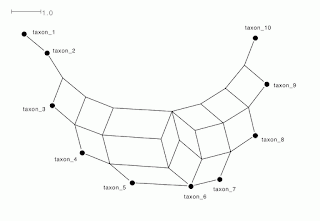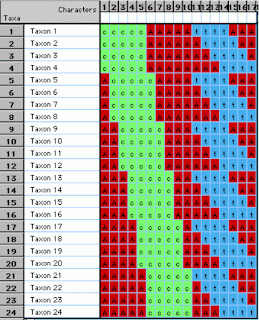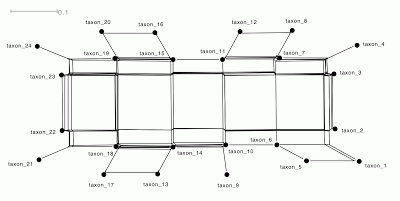## Wednesday, December 19, 2012

### Networks can outperform PCA ordinations in phylogenetic analysis

In a previous blog post I noted that there are potentially Distortions and artifacts in Principal Components Analysis analysis of genome data, in which the Principal Components Analysis (PCA) ordination axes are not independent of each other. This happens when the samples form one or more monotonic gradients, in which case higher-dimensional axes of the ordination plot are curved and twisted relative to the lower axes. The so-called Arch Effect is the best-known example of this problem. The danger is that the ordination diagrams will be interpreted as showing biological patterns when these artifactual patterns are not in the original datasets.

This mathematical artifact can be dealt with by: (i) mathematically correcting the distortion; (ii) using a Non-metric Multidimensional Scaling (NMDS) ordination instead; or (iii) using a phylogenetic network to display the multidimensional data, rather than using an ordination. Here, I illustrate this third possibility.

Network analysis of a 1-dimensional gradient

In the previous blog post I used the following small dataset as an example of a genetic dataset with a 1-dimensional pattern of relationships among the taxa. Here the taxa form a series of overlapping groups, defined by the pattern of Cs.The diagonal dataset.

In the previous post I also illustrated the PCA ordination of this dataset, which shows the classic Arch Effect, rather than the expected linear arrangement of samples in the scatter plot. Below is a table showing whether analyses using two different types of data-display network can effectively display all of the taxon groups for datasets of this type but with varying numbers of taxa. All analyses were performed using the SplitsTree program.

As you can see, the NeighborNet analysis successfully displays all of the relevant bipartitions in all of the datasets. On the other hand, the related Neighbor-Joining tree only succeeds up to a taxon size of six. Six taxa can be represented by a simple linear tree, which can display three non-trivial bipartitions plus six trivial partitions, which is all that is needed when each taxon consists of five Cs. (Note that the NeighborNet and the Median network are identical to this tree, as well, for six taxa or less.)

The next two figures show the NeighborNet graphs for taxon sizes of 10 and 20, respectively, as examples.NeighborNet of the diagonal dataset for n=10.NeighborNet for the diagonal dataset for n=20.

The table also shows that the Median network analysis is only successful for small numbers of taxa. For greater numbers of taxa, some of the bipartitions are not displayed, as listed in the table. For example, for a taxon size of 10, the split {2,3,4,5,6}{1,7,8,9,10} is not displayed, as illustrated in the next figure.Median network for the diagonal dataset for n=10.

For even larger numbers of taxa, the Median network superimposes some of the taxa, even though they have distinct sequences, and thus it cannot display all of the bipartitions, by definition. For example, for a taxon size of 20, taxon_9 and taxon_10 are superimposed, as shown in the next figure.Median network for the diagonal dataset for n=20.

Network analysis of a 2-dimensional gradient

In the previous blog post I used the following dataset as an example of a genetic dataset with a 2-dimensional pattern of relationships among the taxa. Here the taxa form two series of overlapping groups, one defined by the pattern of Cs and the other by the pattern of Ts.The grid dataset.

In the previous post I also illustrated the PCA ordination of this dataset, which shows a complicated 3-dimensional version of the 2-dimensional pattern. On the other hand, the Median network perfectly reproduces the original 2-dimensional grid arrangement of the taxa, as shown next, so that the network diagram is exactly as expected.Median network for the grid dataset.

Sadly, the NeighborNet analysis does not fare quite so well, as it produces a much more complicated arrangement of the taxa. This arrangement does approximately represent the original grid-like pattern contained in the data, but it is presented in an unnecessarily convoluted way.NeighborNet for the grid dataset.

Conclusion

It appears that data-display networks can be used to display multidimensional information, in the same manner as an ordination analysis. These networks try to display all of the relevant bipartitions in the data, rather than simply displaying a scatter plot of the samples.

However, there are considerable differences in the ability of the various network analyses to display the patterns inherent in the original data. In particular, NeighborNet seems to be very effective for displaying 1-dimensional patterns, while Median networks are more effective for 2-dimensional patterns.

Finally, it is worth noting that David Bryant has already thought of comparing NeighborNet with a Multi-dimensional Scaling (MDS) ordination, using an empirical dataset (2006, Radiation and network breaking in Polynesian linguistics. In: Forster P, Renfrew C (eds) Phylogenetic Methods and the Prehistory of Languages. McDonald Institute Press, University of Cambridge, pp 111-118). He found that they approximately agreed with each other.